Next: Motion in 1 dimension Up: Introduction Previous: Worked example 1.2: Tire

## Worked example 1.3: Dimensional analysis

Question: The speed of soundin a gas might plausibly depend on the pressure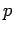, the density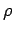, and the volumeof the gas. Use dimensional analysis to determine the exponents,, andin the formula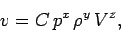where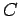is a dimensionless constant. Incidentally, the mks units of pressure are kilograms per meter per second squared.

Answer: Equating the dimensions of both sides of the above equation, we obtain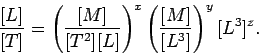A comparison of the exponents of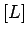,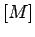, and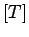on either side of the above expression yields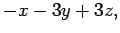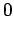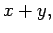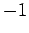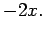The third equation immediately gives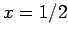; the second equation then yields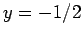; finally, the first equation gives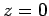. Hence,Next: Motion in 1 dimension Up: Introduction Previous: Worked example 1.2: Tire
Richard Fitzpatrick 2006-02-02